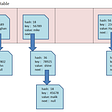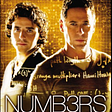# What is the Golden Ratio?

You know you’re truly geeking out when you’re gushing about how beautiful a number is, but hey this number is pretty special ;)

The Golden Ratio has been heralded as the most beautiful ratio in art and architecture for centuries. From the Parthenon to Salvador Dali’s The Sacrament of the Last Supper the Golden Ratio has been found lurking in some of the world’s most celebrated creations.

Now whether you believe this divine proportion is truly a mark of beauty or simply selection bias is up to you, but without a doubt it is one of the most intriguing numbers in existence.

# Phi is Golden

Represented by the greek letter phi (φ), the Golden Ratio is the irrational value:

# Euclid and the Golden Ratio

In book 6 of The Elements, Euclid gives us the definition of the Golden Ratio.

He instructs us to take a line segment and divide it into two smaller segments such that the ratio of the whole line segment (a+b) to segment a is the same as the ratio of segment a to segment b, like this:

Or equivalently as a proportion:

(You geometry buffs out there probably recognize that a is the geometric mean of a+b and b 🙌🏼 )

# The Golden Rectangle

The Golden Ratio is most commonly represented as the Golden Rectangle, a rectangle with side-length ratio of 1.618:1.

Golden Rectangles also have the property that if you cut off a square, you’ll be left with another Golden Rectangle.

# Solving the Golden Proportion

To find where the value 1.618034… comes from we must solve the proportion. For simplicity assume b=1 and a=x so that you may solve for x.

## Step 1

Take the cross products.

## Step 2

Subtract x+1 to set the equation equal to zero.

We now have a standard quadratic with a=1, b=-1, and c=-1.

## Step 3

Plug these values into the quadratic formula and solve.

Since we’re working with lengths, we need only the positive solution.

And there it is! The Golden Ratio, as promised!

For good measure, plug in a=1.618 and b=1 to confirm the proportion holds.

## Notice anything interesting about (1.618 + 1)/1.618 = 1.618?

We can write the Golden Ratio in terms of itself! Which is totally awesome.

Or equivalently,

Now let’s get crazy. Substitute φ=1 + 1/φ for φ in the denominator.

Whoa, that’s cool! Let’s do it again!

We could keep on doing this forever. Which is pretty spectacular. Turns out the Golden Ratio can be written as an infinite continued fraction.

# Finding Fibonacci

We can use the continued fraction to approximate the Golden Ratio and uncover an interesting relationship with the Fibonacci Sequence.

## Step 1

To start out we’re going to alter our continued fraction a little.

Instead of writing the formula nested in itself, we’ll add subscripts to indicate that the next value (φ_n+1) can be generated from the previous value (φ_n).

Since this is an infinite continued fraction, as n increases, the approximation gets closer to the true value of φ.

## Step 2

Define φ_0 = 1. To find φ_1 plug in n=0.

## Step 3

Repeat the process to find φ_2 with n=1, since φ_2 = φ_1+1. Use the result from Step 2 for φ_1.

## Step 4

Keep on repeating this process.

## Step 5

Check it out. There’s the Fibonacci Sequence! Each approximation is the ratio of two adjacent Fibonacci numbers. We no longer need to go through the hassle of plugging values into the continued fraction, we can simply divide successive terms of the Fibonacci Sequence.

As we move forward with each calculation, we find that our approximation of the Golden Ratio is getting closer and closer to its true value.

In fact, the limit of the F(n+1)/F(n) as n → ∞ (where F(n) and F(n+1) represents the nth and nth plus 1 terms in the Fibonacci sequence) converges to φ.

Visually, we can see how the Fibonacci Sequence generates rectangles closer and closer to the coveted Golden Rectangle.

While the design world may be arguing over whether the Golden Ratio is folklore or not, I think it’s safe to say that the Golden Ratio is mathematically intriguing nonetheless.

## ❤ STAY CONNECTED ❤

Stay up-to-date with everything Math Hacks is up to!

--

--

--

## More from Math Hacks

Tutorials with a fresh perspective.

## Automating Snippets in Visual Studio Code with Python## PIXIU token emission rate## Create a Lambda Function With S3 as the Trigger in Cloud9 Using SAM## Rehashing HashMap## A Day In My (New) Programmer Life## Yabai — MacOS Tile Window Manager## How to Run a Hackathon## Building Enterprise Real-Time ETL systems using Kafka Streams — Part 2## Brett Berry

Check out my YouTube channel “Math Hacks” for hands-on math tutorials and lots of math love ♥️

## More from Medium## Electrodynamics: The Unification of Electricity and Magnetism